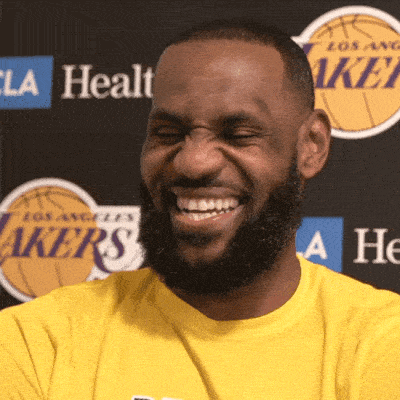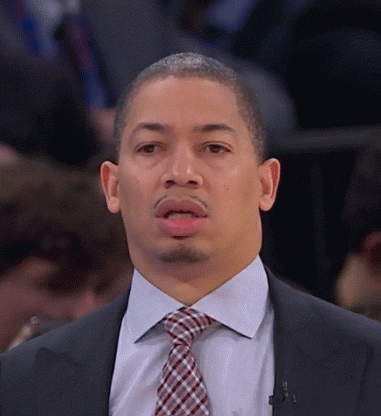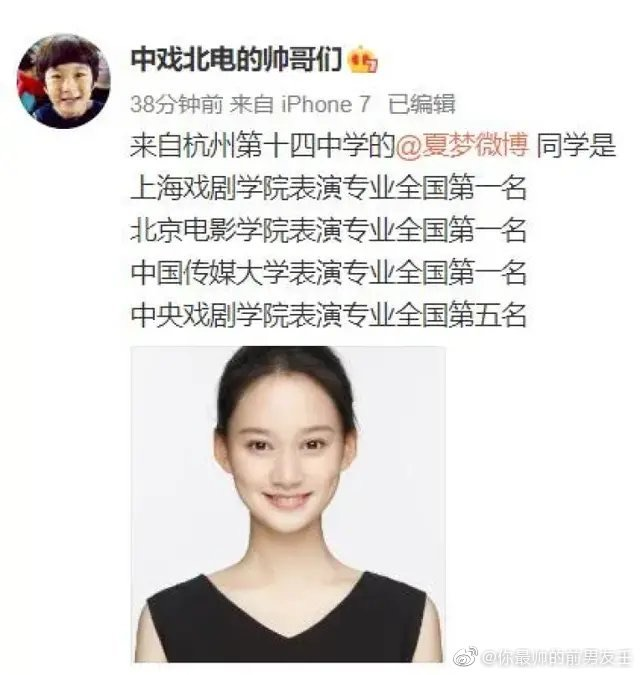NBA英雄

NBA范特西

# 这个艺考生全国第二是什么水平 大家怎么看

559回复/ 501075644 浏览sport346(11级)楼主2022-05-19 14:18:10[图片][图片][图片][图片]100/60=1.666*53.8=89.666
100/80=1.25*71=88.75
100/20*16=80
100/40*34.8=87

100/60=1.666*53.8=89.666
100/80=1.25*71=88.75
100/20*16=80
100/40*34.8=87

Re：这个艺考生全国第二是什么水平 大家怎么看